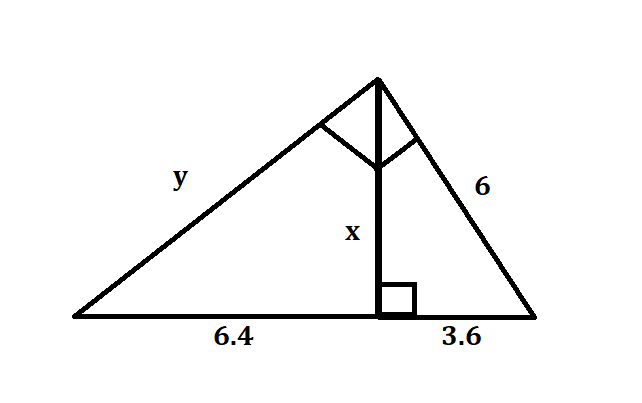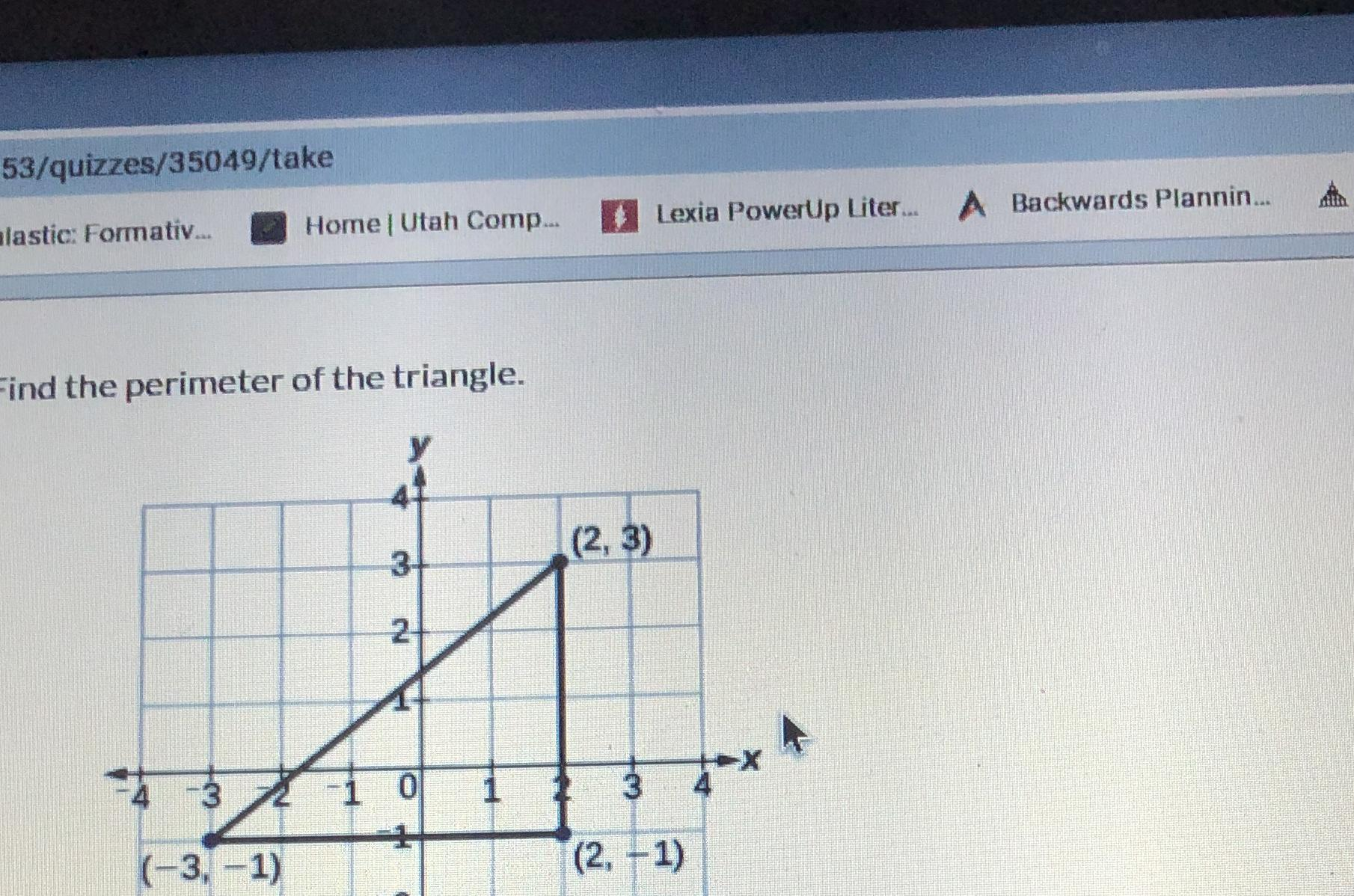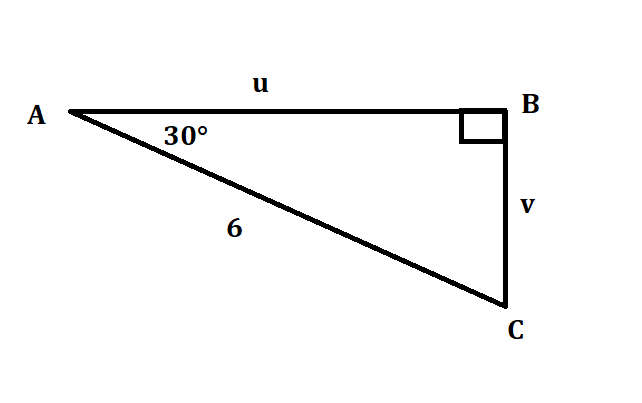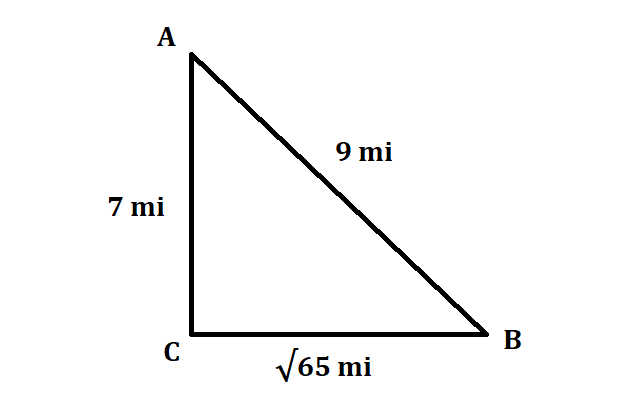# Get help with right triangles and trigonometry

Recent questions in Right triangles and trigonometryDolly Robinson 2021-05-30 Answered

### At what point do the curves ${r}_{1}\left(t\right)=t,4-t,35+{t}^{2}$ and ${r}_{2}\left(s\right)=7-s,s-3,{s}^{2}$ intersect? (x,y,z)= Find angle of intersection, $\theta$, correct to the nearest degree.Chesley 2021-05-19 Answered

### Find the point on the line $y=5x+3$ that is closet to the origin.lwfrgin 2021-05-18 Answered

### In triangle DEF, side E is 4 cm long and side F is 7 cm long. If the angle between sides E and F is 50 degrees, how long is side D?Bergen 2021-05-14 Answered

### Aidan knows that the observation deck on the Vancouver Lookout is 130 m above the ground. He measures the angle between the ground and his line of sight to the observation deck as ${77}^{\circ }$. How far is Aidan from the base of the Lookout to the nearest metre?Rivka Thorpe 2021-05-08 Answered

### The leg of a right triangle that lies on one ray of angle $\theta$ is called the ? leg, and the leg that lies across triangle from $\theta$ is called the ? leg.iohanetc 2021-03-12 Answered

### Aidan knows that the observation deck on the Vancouver Lookout is 130 m above the ground. He measures the angle between the ground and his line of sight to the observation deck as ${77}^{\circ }$. How far is Aidan from the base of the Lookout to the nearest metre?Falak Kinney 2021-03-09 Answered

### Determine whether the set have a subset relationship. Are the two sets disjoint or equivalent? Do the set intersect? I={isosceles triangles}, R={right triangles}zi2lalZ 2021-03-09 Answered

### Find the values of the variables in each right triangle.Bergen 2021-03-08 Answered

### Diameter is 135 meters and makes one revolution every 30 minutes. Find a sinsuoid which models the height h of the passenger above the ground in meters t minutes after they board.vestirme4 2021-03-08 Answered

### Peter says the triangle in not an equilateral triangle because neither all the sides nor all the angles are marked as congruent. Do you agree with peter?floymdiT 2021-03-08 Answered

### Rewrite the statements in if-then form. Having two ${45}^{\circ }$ angles is a sufficient condition for this triangle to be a right triangle.defazajx 2021-03-07 Answered

### The following are the dimensions of a few rectangles. Find the are of the two right triangles that are cut from the rectangles using the formmula of the area of a triangle. A. Lenght= 13.5 m, Breadth=10.5 m B. Lenght=18 m, Breadth=8 cm C. Lenght=1.5 m, Breadth=30 cmAlbarellak 2021-03-07 Answered

### Find the solution $\mathrm{sin}Y=\mathrm{cos}\left(Y+20°\right)$Sinead Mcgee 2021-03-07 Answered

### Given $△ABC\cong △DEF$, name all of the corresponding parts you could prove congruent using CPCTCabondantQ 2021-03-05 Answered

### Find the perimeter of the triangle.Clifland 2021-03-05 Answered

### Label the words of this 30,60,90 right triangle $SL,2SL,\phantom{\rule{1em}{0ex}}\text{and}\phantom{\rule{1em}{0ex}}SL\sqrt{2}$generals336 2021-03-02 Answered

### The lengths of the diagonals of a rectangle are represented by 8x + 3 feet and 4x + 7 feet. Find the length of each diagonal.ringearV 2021-03-02 Answered

### Is this triangle a right triangleka1leE 2021-03-02 Answered

### The converse of the Pythagorean theorem is also a true statement: If the sum of the squares of the lengths of two sides of a triangle is equal to the square of the length of the third side, then the triangle is a right triangle. Use the distance formula and the Pythagorean theorem to determine whether the set of points could be vertices of a right triangle. (-4,3), (0,5), and (3,-4)Wribreeminsl 2021-03-01 Answered